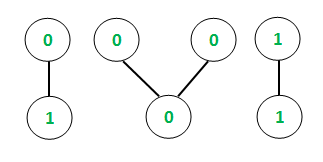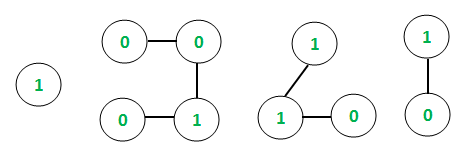# Maximum decimal equivalent possible among all connected components of a Binary Valued Graph

Given a binary valued Undirected Graph with V vertices and E edges, the task is to find the maximum decimal equivalent among all the connected components of the graph. A binary valued graph can be considered as having only binary numbers (0 or 1) as the vertex values.

Examples:

Input: E = 4, V = 7Output: 3
Explanation:
Decimal equivalents of the connected components are as follows:
[0, 1] : Maximum possible decimal equivalent = 2 [(10)2]
[0, 0, 0] : Maximum possible decimal equivalent = 2
[1, 1] : Maximum possible decimal equivalent = 3
Hence, Maximum decimal equivalent of all components = 3

Input: E = 6, V = 10Output: 8
Explanation:
Connected Components and decimal equivalent are as follows:
 : Maximum possible decimal equivalent = 2
[0, 0, 1, 0] : Maximum possible decimal equivalent = 8 [(1000)2]
[1, 1, 0] : Maximum possible decimal equivalent = 6
[1, 0] : Maximum possible decimal equivalent = 2
Hence, Maximum decimal equivalent of all components = 8

Approach:

• The idea is to use Depth First Search Traversal to keep track of the connected components in the undirected graph as explained in this article.
• For each connected component, the binary string is stored and the equivalent decimal value is calculated.
• A global maximum is set that is compared to maximum decimal equivalent obtained after every iteration to get the final result.

Below is the implementation of the above approach:

## C++

 `// C++ Program to find ` `// maximum decimal equivalent among ` `// all connected components ` ` `  `#include ` ` `  `using` `namespace` `std; ` ` `  `// Function to traverse the undirected ` `// graph using the Depth first traversal ` `void` `depthFirst(``int` `v, vector<``int``> graph[], ` `                ``vector<``bool``>& visited, ` `                ``vector<``int``>& storeChain) ` `{ ` `    ``// Marking the visited ` `    ``// vertex as true ` `    ``visited[v] = ``true``; ` ` `  `    ``// Store the connected chain ` `    ``storeChain.push_back(v); ` ` `  `    ``for` `(``auto` `i : graph[v]) { ` `        ``if` `(visited[i] == ``false``) { ` ` `  `            ``// Recursive call to ` `            ``// the DFS algorithm ` `            ``depthFirst(i, graph, ` `                       ``visited, storeChain); ` `        ``} ` `    ``} ` `} ` ` `  `// Function to return decimal ` `// equivalent of each connected ` `// component ` `int` `decimal(``int` `arr[], ``int` `n) ` `{ ` `    ``int` `zeros = 0, ones = 0; ` ` `  `    ``// Storing the respective ` `    ``// counts of 1's and 0's ` `    ``for` `(``int` `i = 0; i < n; i++) { ` `        ``if` `(arr[i] == 0) ` `            ``zeros++; ` `        ``else` `            ``ones++; ` `    ``} ` ` `  `    ``// If all are zero then ` `    ``// maximum decimal equivalent ` `    ``// is also zero ` `    ``if` `(zeros == n) ` `        ``return` `0; ` ` `  `    ``int` `temp = n - ones; ` `    ``int` `dec = 0; ` ` `  `    ``// For all the 1's, calculate ` `    ``// the decimal equivalent by ` `    ``// appropriate multiplication ` `    ``// of power of 2's ` `    ``while` `(ones--) { ` `        ``dec += ``pow``(2, temp); ` `        ``temp++; ` `    ``} ` `    ``return` `dec; ` `} ` ` `  `// Function to find the maximum ` `// decimal equivalent among all ` `// connected components ` `void` `decimalValue( ` `    ``vector<``int``> graph[], ``int` `vertices, ` `    ``vector<``int``> values) ` `{ ` `    ``// Initializing boolean array ` `    ``// to mark visited vertices ` `    ``vector<``bool``> visited(10001, ``false``); ` ` `  `    ``// maxDeci stores the ` `    ``// maximum decimal value ` `    ``int` `maxDeci = INT_MIN; ` ` `  `    ``// Following loop invokes ` `    ``// DFS algorithm ` `    ``for` `(``int` `i = 1; i <= vertices; i++) { ` `        ``if` `(visited[i] == ``false``) { ` ` `  `            ``// Variable to hold ` `            ``// temporary length ` `            ``int` `sizeChain; ` ` `  `            ``// Variable to hold temporary ` `            ``// Decimal values ` `            ``int` `tempDeci; ` ` `  `            ``// Container to store ` `            ``// each chain ` `            ``vector<``int``> storeChain; ` ` `  `            ``// DFS algorithm ` `            ``depthFirst(i, graph, visited, ` `                       ``storeChain); ` ` `  `            ``// Variable to hold each ` `            ``// chain size ` `            ``sizeChain = storeChain.size(); ` ` `  `            ``// Container to store values ` `            ``// of vertices of individual ` `            ``// chains ` `            ``int` `chainValues[sizeChain + 1]; ` ` `  `            ``// Storing the values of ` `            ``// each chain ` `            ``for` `(``int` `i = 0; i < sizeChain; i++) { ` `                ``int` `temp = values[storeChain[i] - 1]; ` `                ``chainValues[i] = temp; ` `            ``} ` ` `  `            ``// Function call to find ` `            ``// decimal equivalent ` `            ``tempDeci = decimal(chainValues, ` `                               ``sizeChain); ` ` `  `            ``// Conditional to store maximum ` `            ``// value of decimal equivalent ` `            ``if` `(tempDeci > maxDeci) { ` `                ``maxDeci = tempDeci; ` `            ``} ` `        ``} ` `    ``} ` ` `  `    ``// Printing the decimal result ` `    ``// (global maxima) ` ` `  `    ``cout << maxDeci; ` `} ` ` `  `// Driver code ` `int` `main() ` `{ ` `    ``// Initializing graph in the ` `    ``// form of adjacency list ` `    ``vector<``int``> graph; ` ` `  `    ``// Defining the number of ` `    ``// edges and vertices ` `    ``int` `E, V; ` `    ``E = 4; ` `    ``V = 7; ` ` `  `    ``// Assigning the values ` `    ``// for each vertex of the ` `    ``// undirected graph ` `    ``vector<``int``> values; ` `    ``values.push_back(0); ` `    ``values.push_back(1); ` `    ``values.push_back(0); ` `    ``values.push_back(0); ` `    ``values.push_back(0); ` `    ``values.push_back(1); ` `    ``values.push_back(1); ` ` `  `    ``// Constructing the ` `    ``// undirected graph ` `    ``graph.push_back(2); ` `    ``graph.push_back(1); ` `    ``graph.push_back(4); ` `    ``graph.push_back(3); ` `    ``graph.push_back(5); ` `    ``graph.push_back(4); ` `    ``graph.push_back(7); ` `    ``graph.push_back(6); ` ` `  `    ``decimalValue(graph, V, values); ` `    ``return` `0; ` `} `

Output:

```3
```

Complexity analysis:
Time Complexity: O(V2)
The DFS algorithm takes O(V + E) time to run, where V, E are the vertices and edges of the undirected graph. Further, the decimal equivalent is found at each iteration that takes an additional O(V) to compute and return the result. Hence, the overall complexity is O(V2)My Personal Notes arrow_drop_upCheck out this Author's contributed articles.

If you like GeeksforGeeks and would like to contribute, you can also write an article using contribute.geeksforgeeks.org or mail your article to contribute@geeksforgeeks.org. See your article appearing on the GeeksforGeeks main page and help other Geeks.

Please Improve this article if you find anything incorrect by clicking on the "Improve Article" button below.